# Solving Two-Step Equations With Context [Part 2]

Visual math talk prompts with context used to help emerge the writing and solving of two-step algebraic equations involving partitive division.

## In This Set of Math Visual Prompts…

Students will explore modelling and solving two-step algebraic equations involving multiplication and division.

## Intentionality…

This set of visual math talk prompts is taken from the Math Talk section of Day 4 in the Make Math Moments Problem Based Unit called Planting Flowers Revisited. The purpose of the Math Talk is to reinforce key concepts and big ideas from this problem based math unit including:

• There are two types of division;
• Partitive division is when the total quota is known (the dividend), and the number of parts or groups (the divisor) is known;
• Partitive division reveals a rate;
• In partitive division, the dividend and the divisor often have different units;
• The dividend from any division sentence can be decomposed into smaller parts to allow for friendlier division by the divisor. This strategy is known as partial quotients. (i.e.: 85 ÷ 5 = 45 ÷ 5 + 40 ÷ 5 = 9 + 8 = 17);
• Division is the inverse operation of multiplication;
• Addition is the inverse operation of addition;
• Variables are used to represent changing or unknown quantities;
• When solving an equation where the coefficient of the unknown variable is not equal to 1, division is required to determine the value of the unknown variable and the context of the problem determines which type of division is required.
• A two-step equation is an algebraic equation that takes you two steps to solve.

Unsure about some of the terminology listed above?

Learn more about multiplicative thinking, rates, ratios and the roadmap to proportional relationships through the course: The Concept Holding Your Students Back.

## Preparing to Facilitate

Present the following equations one at a time. Encourage students to describe a context that could be represented by each. All of today’s equations and contexts should be partitive. Meaning, the number of groups (parts) is known, the rate is unknown.

For example:

Luisa has 4 large containers with an equal number of monarch caterpillars in each. She also has two swallowtail caterpillars in a smaller container. If she has 30 caterpillars altogether, how many caterpillars are in each of the large containers?

Write an equation and solve using a model of your choice.

Students should use a model of their choice to solve and/or represent their thinking.

Four (4) additional visual math prompts are shared in the Teacher Guide from Day 4 of the Planting Flowers Revisited problem based math unit

Although you could lead this math talk by orally sharing the context and representing student thinking on a chalkboard/whiteboard, consider leveraging the following visual math talk prompt videos to ensure accessibility for all students. We would recommend that you still model student thinking to ensure student voice is honoured prior to sharing the visual silent solution shared in the visual prompt video.

Visual Math Talk Prompt #1

Show the visual math talk prompt video and be ready to pause once prompted.

In the video, students are presented with visuals and a text prompt to set the context involving an equal quantity of caterpillars stored in large containers plus an additional two caterpillars in a smaller container.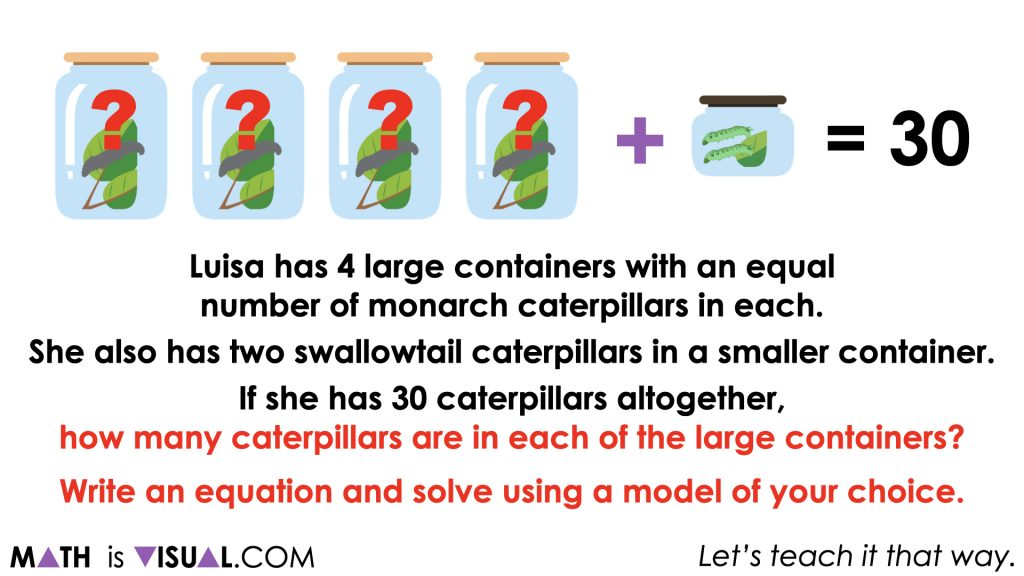You’ll notice that this first prompt attempts to make this context very visual and scaffolds students toward an algebraic equation that could be used to represent this situation, 4m + 2 = 30.

It is worth noting that this context is going to require the use of partitive division as the unknown variable represents the rate of caterpillars per large container while the number of large containers is known.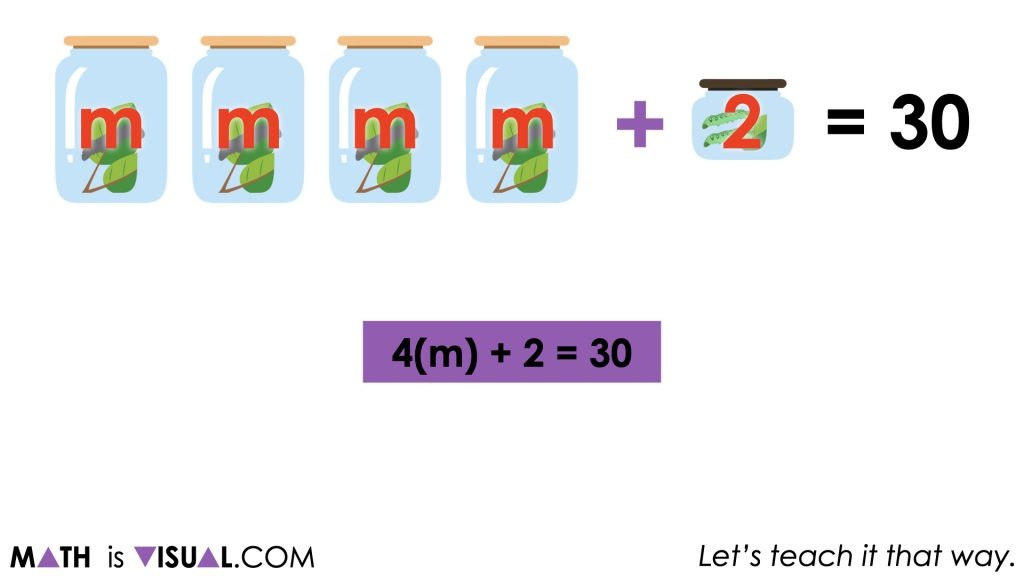Some students might immediately begin using trial and error to determine the value of m. For example, some students might try m = 5 and will skip count to 20 (or multiply 4 large containers by 5 caterpillars) plus 2 caterpillars is 22. Therefore, they might adjust up until they land on the value of m that will result in a total of 30 caterpillars.

Other students might leverage a linear model like a stacked bar model beginning with 30 caterpillars and working backwards to reveal how many caterpillars must be in the 4 large containers. By subtracting the two additional caterpillars, students will quickly realize that there must be 28 caterpillars divided equally across the 4 large containers.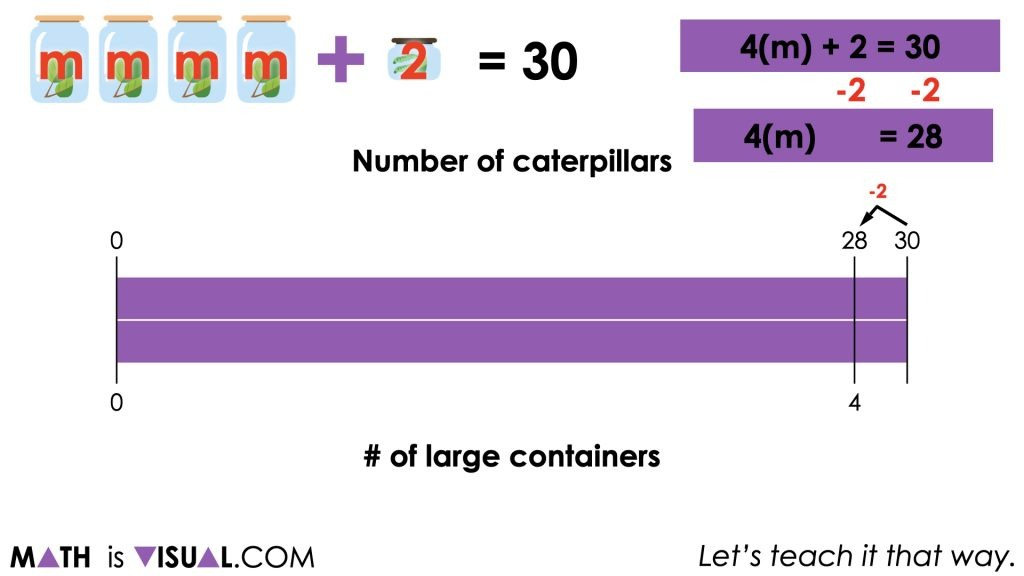Now that the 2 additional caterpillars have been removed from the equation, we now have a simple one step equation involving partitive division to solve. Some students might choose to scale down by halving twice, or other students might immediately partition the quantity into 4 groups representing the 4 large containers.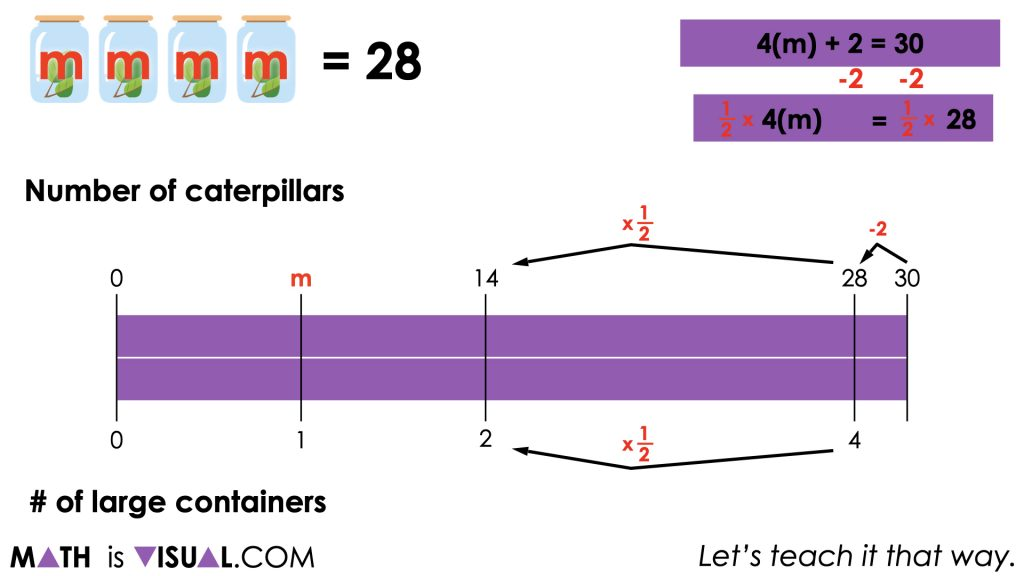Ensure that students are able to connect how multiplying by 1 fourth (or by 1 half and again by 1 half) is equivalent to dividing by 4. This idea can be helpful for students to solidify their understanding of how dividing by a quantity is equivalent to multiplying by the reciprocal.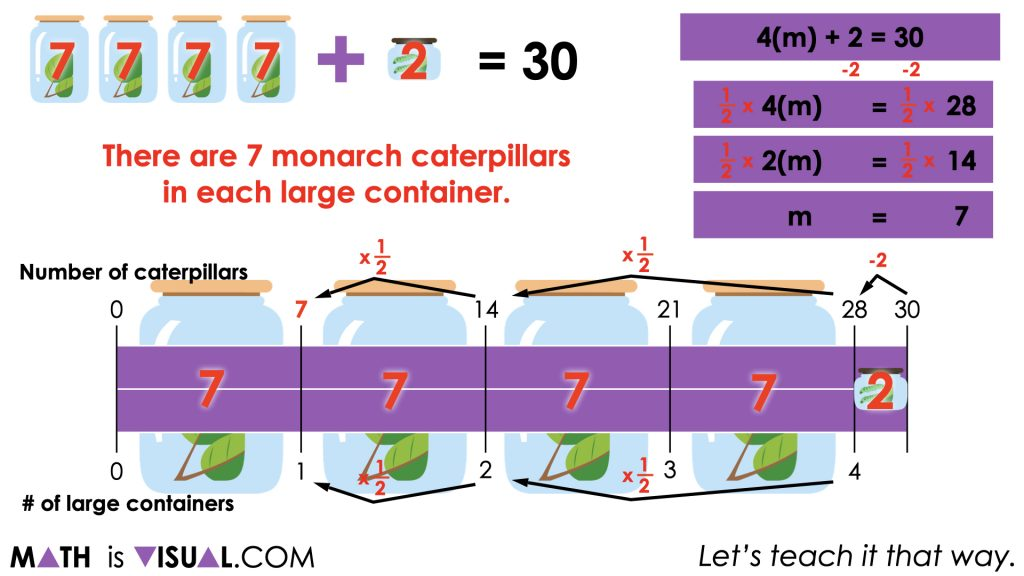Facilitator Note:

Be sure to ask students about what they notice when they analyze the equation 4(m) + 2 = 30 and the operations they used to solve for the unknown number of caterpillars per large container, m. Some students may be able to recognize that we are using opposite operations to undo or work backwards to find the value of m. Ensure this is explicitly highlighted.

Visual Math Talk Prompt #2

In this next visual math talk prompt, we are encouraging students to start with an equation and attempt to craft a scenario using the same context.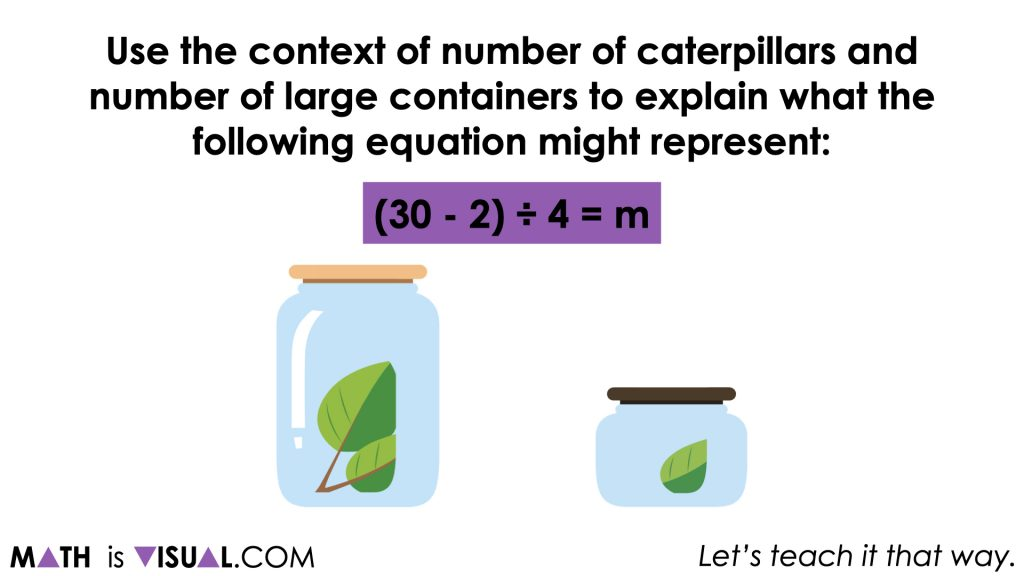Some students may quickly realize that this equation is equivalent to the equation from the first visual math talk prompt and we can now highlight the use of opposite operations leveraged in the previous prompt (subtracting 2 caterpillars and then dividing by 4 large containers).

Encourage students to articulate what each of the quantities in this equation could represent in this scenario.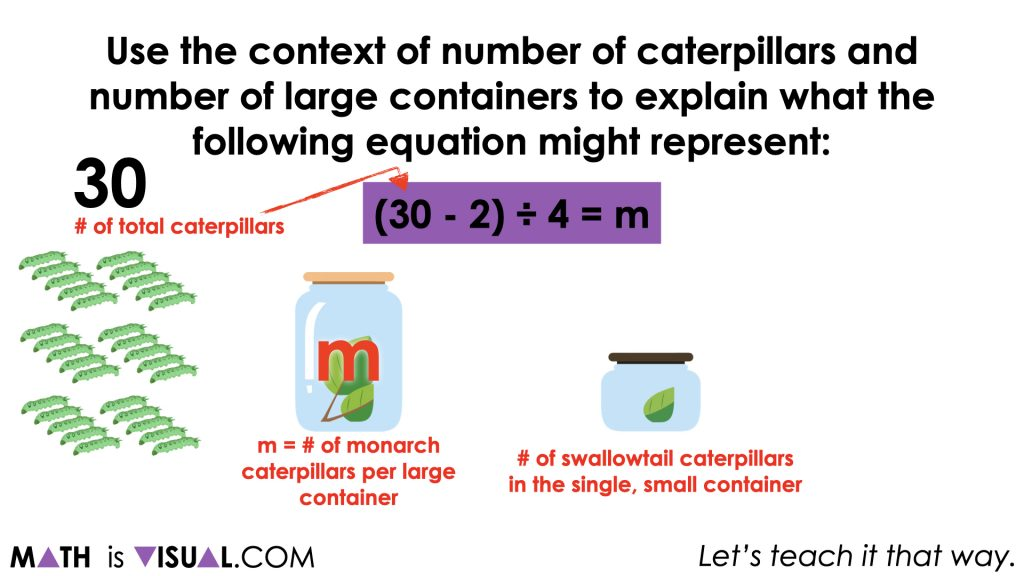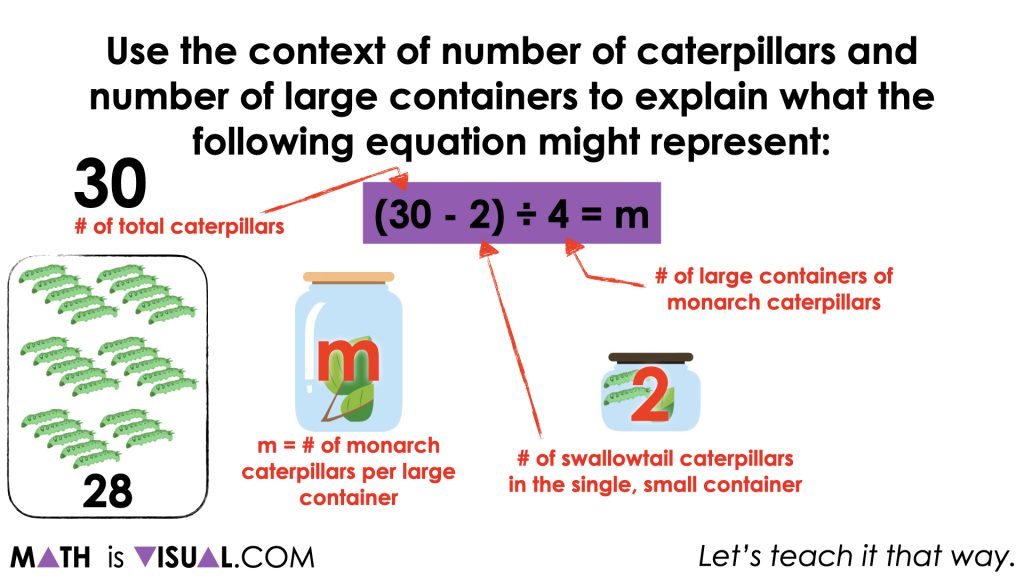In the visual math talk prompt video, you’ll see the 30 caterpillars being partitioned by subtracting 2 caterpillars to be stored in the single small container and the remaining 28 caterpillars fair shared (divided partitively) across 4 large containers.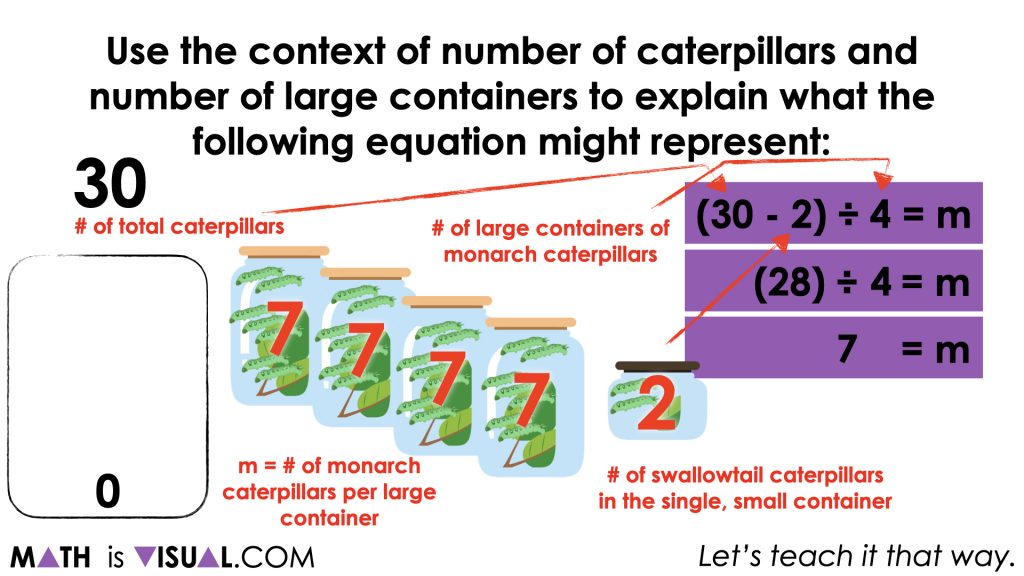We can also leverage our stacked bar model from the previous prompt to show how this equivalent equation can be solved for m by performing the same operations.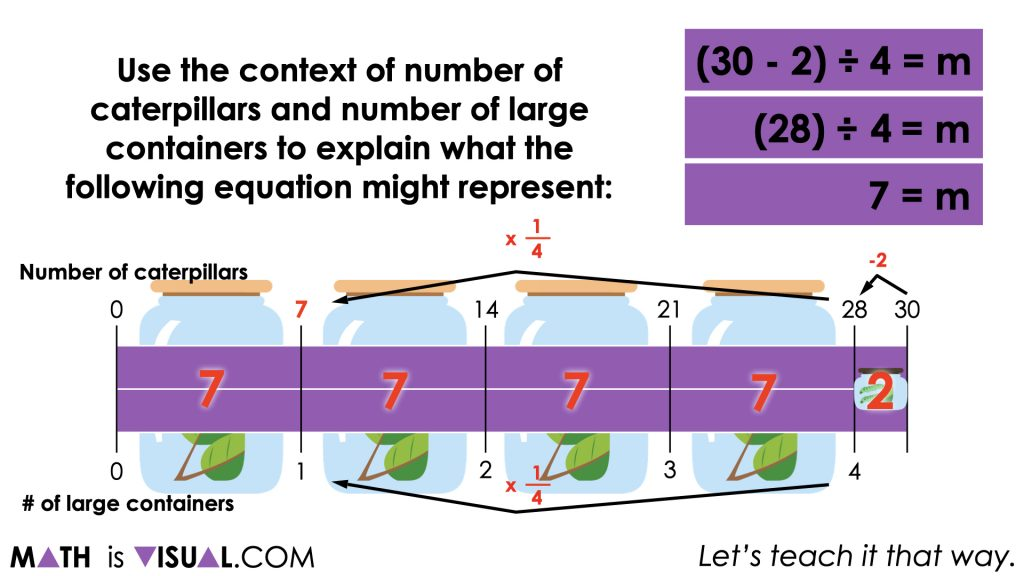## Want to Explore These Concepts & Skills Further?

Four (4) additional math talk prompts are available in Day 4 of the Planting Flowers Revisited problem based math unit that you can dive into now.

Why not start from the beginning of this contextual 5-day unit of real world lessons from the Make Math Moments Problem Based Units page.

Did you use this in your classroom or at home? How’d it go? Post in the comments!

Math IS Visual. Let’s teach it that way.